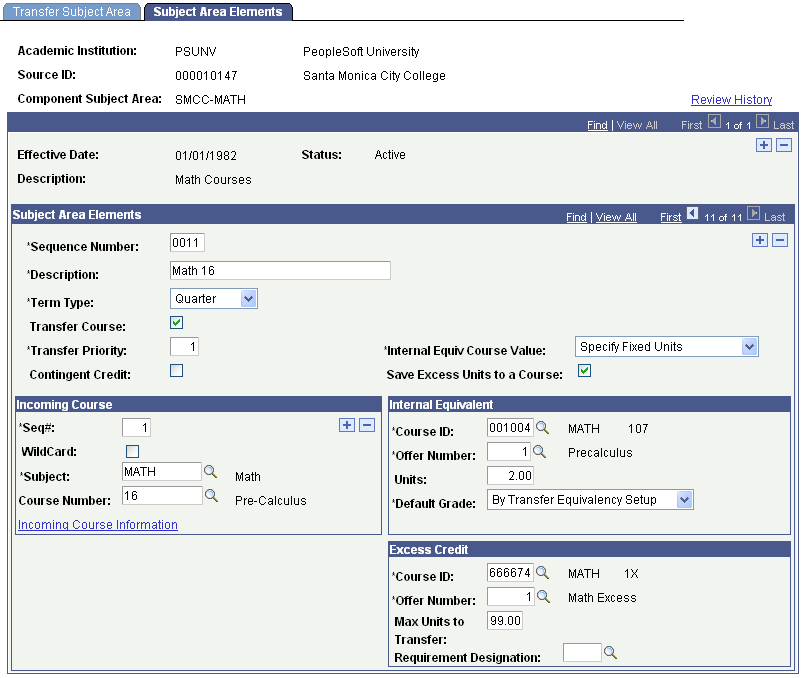# Reviewing Examples of Course Equivalencies

This section provides examples of the following course equivalencies:

• Many-to-one.

• Many-to-many.

• Course rejection.

• Multiple equivalencies for the same course.

• Excess credit.

These examples demonstrate some of the ways that you can set up the Subject Area Elements page for course equivalencies.

Image: Example of many-to-one course equivalency

This example illustrates a many-to-one course equivalency: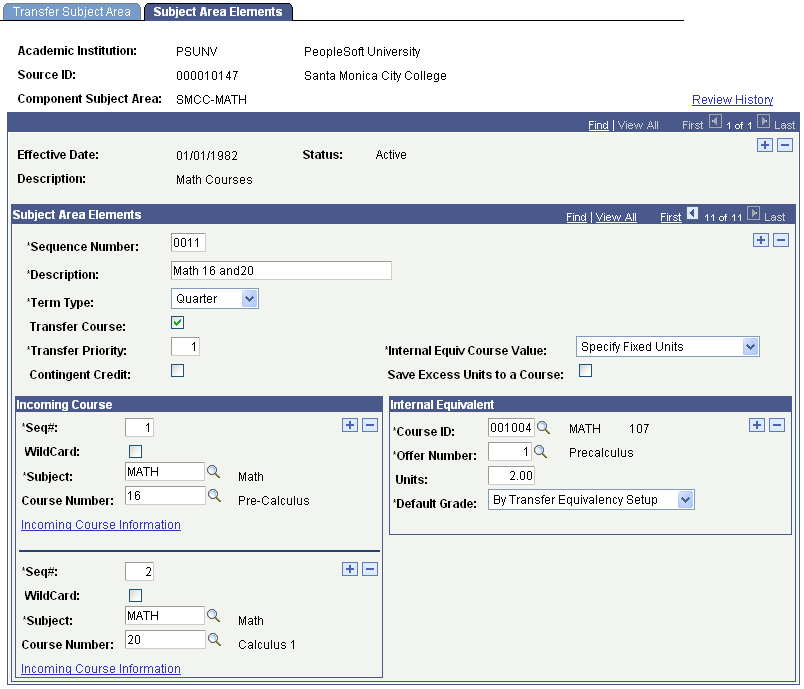When the Transfer Credit process applies this rule, it uses the transfer grade value defined on the Academic Program Table component for the academic program specified on the transfer credit model.

Image: Example of many-to-many course equivalency

This example illustrates a many-to-many course equivalency: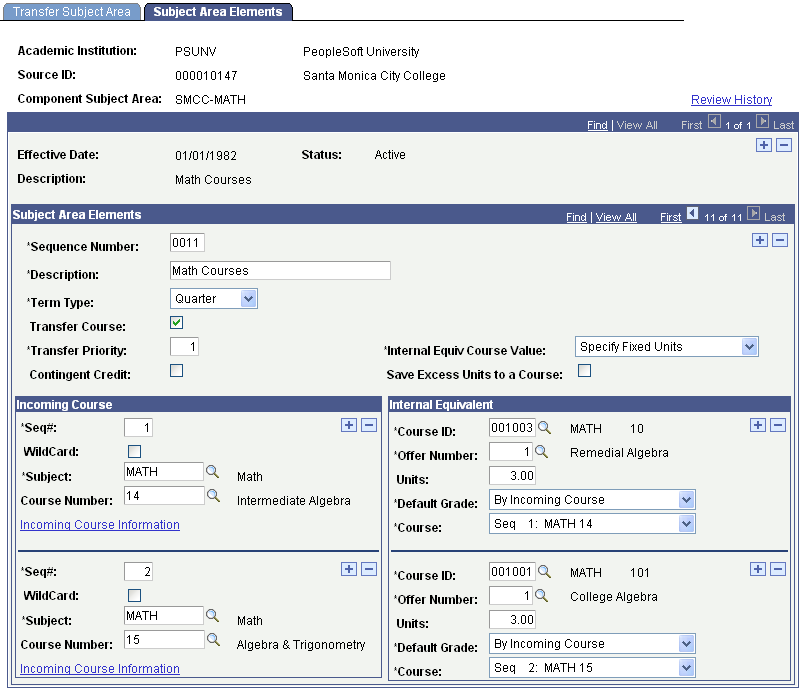When the Transfer Credit process applies this rule, it uses the transfer grade for MATH 14 for one course and the transfer grade for MATH 15 for the other course.

To reject a course, clear the Transfer Course check box. The Internal Equivalent group box fields become unavailable.

Image: Example of a course rejection equivalency

This example illustrates a course rejection equivalency: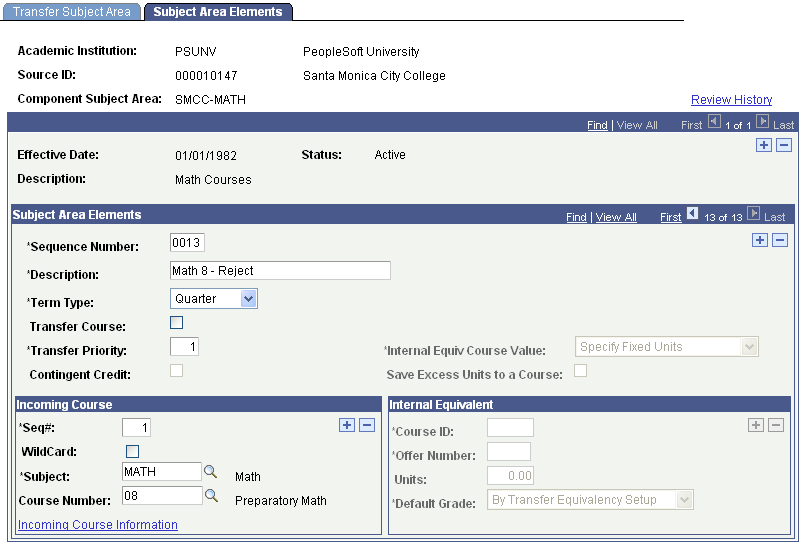The following examples show multiple equivalencies for the same course.

For Santa Monica City College, the student's transfer course articulates to different math courses at PSUNV, depending on the number of units that a student takes for Math 20. In this example, there are two component rules—one for Math 20 taken between one and two units and another for Math 20 taken between three and four units.

Image: Example of multiple equivalencies for the same course (1 of 4)

This example illustrates the one to two unit equivalency: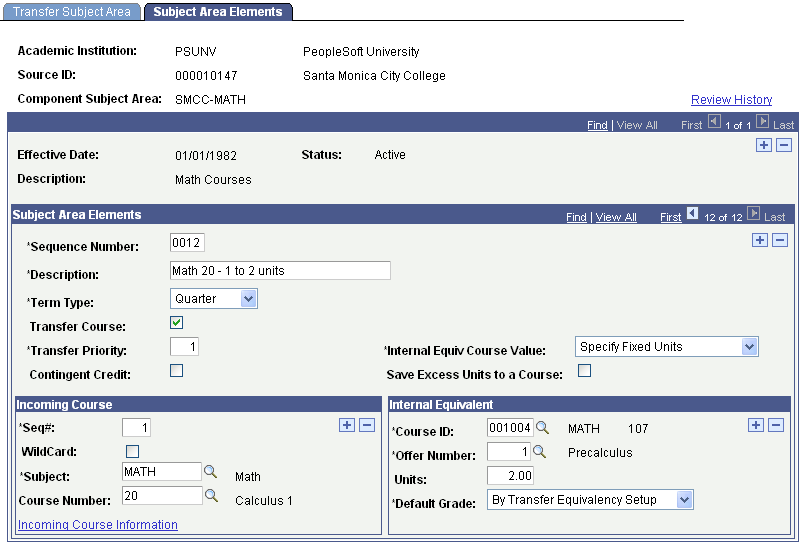The incoming course maps to Pre-calculus.

Image: Example of multiple equivalencies for the same course (2 of 4)

This example illustrates the Incoming Course Information page for the one-to-two unit equivalency: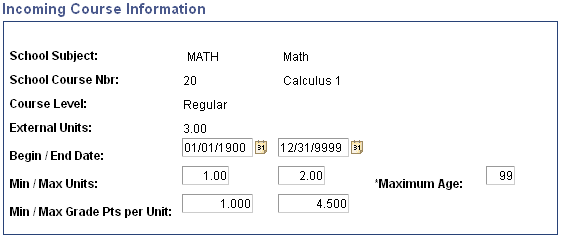The minimum and maximum units are 1.00 and 2.00, respectively.

Image: Example of multiple equivalencies for the same course (3 of 4)

This example illustrates the three-to-four unit equivalency: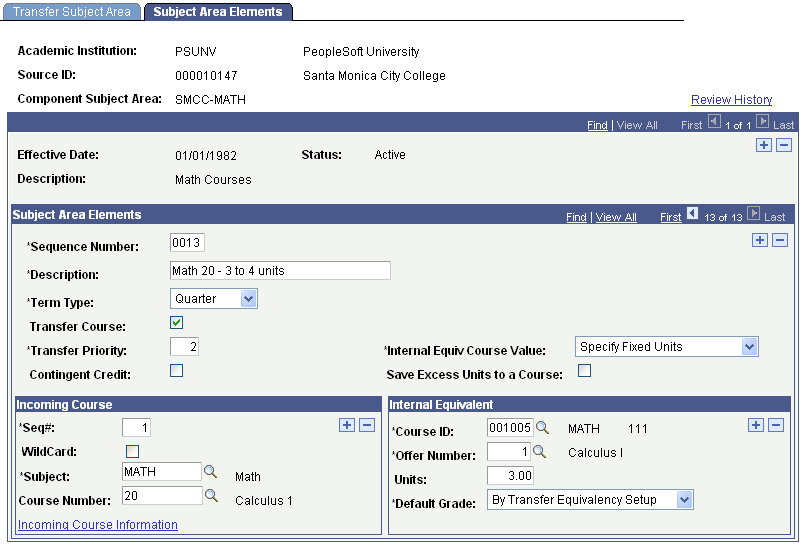The incoming course maps to a Calculus 1 instead of Pre-calculus.

Image: Example of multiple equivalencies for the same course (4 of 4)

This example illustrates the Incoming Course Information page for the three-to-four unit equivalency: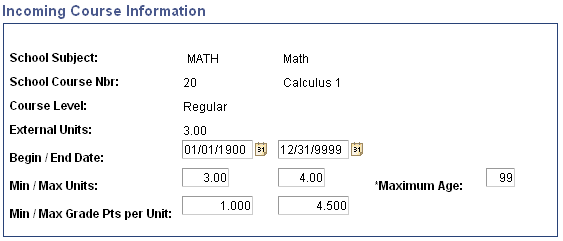The minimum and maximum units are 3.00 and 4.00, respectively.

Excess credit occurs when the external course is worth more units than the internal equivalent course. Identify an existing course or create a new course to manage excess credit.

Image: Example of an excess credit course equivalency

This example illustrates an excess credit course equivalency: